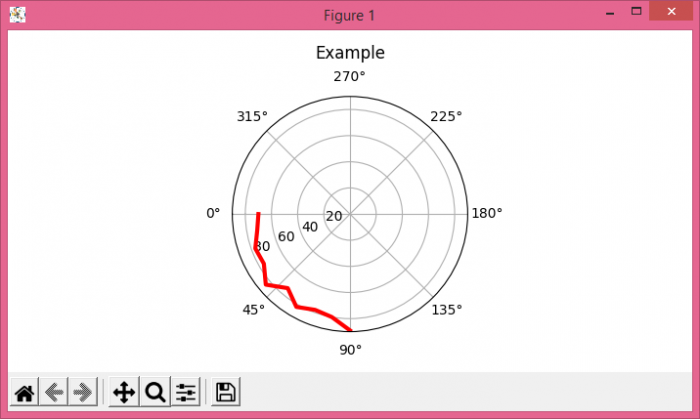# Rotate theta=0 on a Matplotlib polar plot

To set theta=0 on a matplotlib polar plot, we can take the following steps −

• Create random theta in the range of 0 to 100; convert them into radian.

• Using set_theta_zero_location() method, we can set the location of theta to 0.

• Plot theta_in_rad and data_r using plot() method.

• Set the title of the plot using title() method.

• To display the figure, use show() method.

## Example

import numpy as np
import matplotlib.pyplot as plt
import random
plt.rcParams["figure.figsize"] = [7.00, 3.50]
plt.rcParams["figure.autolayout"] = True
theta_in_rad = [float(i) * np.pi / 180.0 for i in range(0, 100, 10)]
data_r = random.sample(range(70, 90), 10)
ax = plt.subplot(111, polar=True)
ax.set_theta_zero_location("W")
ax.set_title("Example", va='bottom')
plt.show()

## OutputUpdated on: 08-May-2021

630 Views